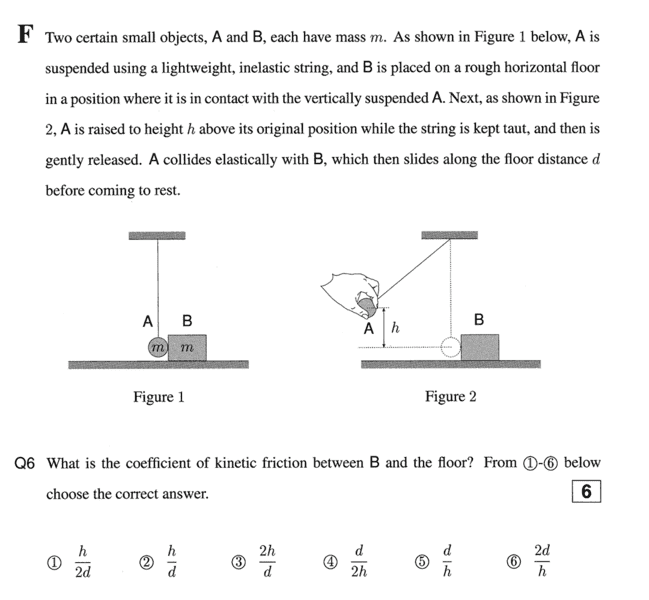# Elastic collision problem (μk) is the target variable

• PedroPicapiedra

## Homework Statement

Two certain small objects A and B, each have mass m. A is suspended using a lightweight, inelastic string, and B is placed on a rough horizontal floor in a position where it is in contact with the vertically suspended A. Next, A is raised to height h above its original position while the string is kept taut, and then is gently released. A collides elastically with B, which then slides along the floor distance d before coming to a rest## Homework Equations

(mA)(vA1)+(mB)(vB1) = (mA)(vA2)+(mA)(vA2)
K1 + U1 + W other = K2 + U2

## The Attempt at a Solution

To my understanding, in collision problems the momentum is always conserved. However, energy is not necessarily conserved.
So I used the momentum equation and and inserted the variables. Since object B is at rest before the collision, and object A is at rest after the collision; I got rid of (mB)(vB1) and (mA)(vA2).
Therefore:
(mA)(vA1) = (mB)(vB2) since both masses are equal I got that both velocities are equal.
(vA1) = (vB2)

Then I use the energy equation where

K1 = 0
U1 = mgh
W other = (Ff)(d) = (μk)(n) = (μk)(-mg)
K2 = 1/2mv^2
U2 = 0

Thus
mgh - (μk)(mg)(d) = 1/2mv^2
gh - (μk)(g)(d) = 1/2v^2
h - (μk)(d) = (v^2)/(2g)
(μk) = (2gh - v^2)/(2g)(d)

I don' really know where I went wrong. But I think my energy equation is wrong.

The answer is supposed to be h/d.

Thank you.

#### Attachments

You are correct that A is at rest after the collision. Also, energy is conserved except that energy is lost as B slides along the floor (that energy goes into heat). Write an expression for the total energy just before A is released and another expression for the total energy right after the collision. Then relate the energy lost due to friction to the distance B slides.

## Homework Statement

Two certain small objects A and B, each have mass m. A is suspended using a lightweight, inelastic string, and B is placed on a rough horizontal floor in a position where it is in contact with the vertically suspended A. Next, A is raised to height h above its original position while the string is kept taut, and then is gently released. A collides elastically with B, which then slides along the floor distance d before coming to a rest
View attachment 226460

## Homework Equations

(mA)(vA1)+(mB)(vB1) = (mA)(vA2)+(mA)(vA2)
K1 + U1 + W other = K2 + U2

## The Attempt at a Solution

To my understanding, in collision problems the momentum is always conserved. However, energy is not necessarily conserved.
So I used the momentum equation and and inserted the variables. Since object B is at rest before the collision, and object A is at rest after the collision; I got rid of (mB)(vB1) and (mA)(vA2).
Therefore:
(mA)(vA1) = (mB)(vB2) since both masses are equal I got that both velocities are equal.
(vA1) = (vB2)

Then I use the energy equation where

K1 = 0
U1 = mgh
W other = (Ff)(d) = (μk)(n) = (μk)(-mg)
K2 = 1/2mv^2
U2 = 0

Thus
mgh - (μk)(mg)(d) = 1/2mv^2
gh - (μk)(g)(d) = 1/2v^2
h - (μk)(d) = (v^2)/(2g)
(μk) = (2gh - v^2)/(2g)(d)

I don' really know where I went wrong. But I think my energy equation is wrong.

The answer is supposed to be h/d.

Thank you.
The problem states that the collision is elastic. That means that no energy is lost in the collision, so energy is conserved.
The mass A is not necessarily at rest after the collision, but you can use conservation of energy and conservation of momentum to figure out what its velocity is. In this case, your two wrong assumptions canceled out and you got the right answer anyway. All of A's momentum is transferred to B. That will not happen if the two masses are different or if collision is not head on.

I don't understand what "W other" means, or how you are trying to use it in an energy equation. Energy is lost to friction as B slides to a stop, so you could equate the work the block does against friction to its initial kinetic energy and solve for d.